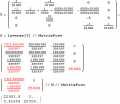# LTSpice simulation of a two-port network.

#### AksharGandhi05

Joined Mar 28, 2020
4
Here's the question : "For the two port network given, obtain the Z-parameters, ABCD (transmission) parameters and h (hybrid) parameters. What should be the value of load resistance connected at Port-2 for maximum power transfer when a voltage source of 25V is connected at Port-1." (Picture attached herewith).

I have done the question by hand, but I'm having trouble writing a netlist for finding answers in the required method. If someone could help me write a netlist, I'l greatly appreciate it. I'm very new to LTSpice and have close to no experience, so some helpful resources to learn LTSpice will also be appreciated.

Values :
R1 = 20k
R2 = 6k
R3 = 25k
K = 13

These are the answers after calculation by hand :
Z - Param :
 z11 = 33.45k z12 = 0 z21 = 29.146k z22 = 25k

H - Param :
 h11 = 33.45k h12 = 0 h21 = -1.16 h22 = 0.04m

Trans - Param :
 t11 = 1.1476 t12 = 28.69k t21 = 0.034m t22 = 0.858

#### The Electrician

Joined Oct 9, 2007
2,866
Here's the question : "For the two port network given, obtain the Z-parameters, ABCD (transmission) parameters and h (hybrid) parameters. What should be the value of load resistance connected at Port-2 for maximum power transfer when a voltage source of 25V is connected at Port-1." (Picture attached herewith).

I have done the question by hand, but I'm having trouble writing a netlist for finding answers in the required method. If someone could help me write a netlist, I'l greatly appreciate it. I'm very new to LTSpice and have close to no experience, so some helpful resources to learn LTSpice will also be appreciated.

Values :
R1 = 20k
R2 = 6k
R3 = 25k
K = 13

These are the answers after calculation by hand :
Z - Param :
 z11 = 33.45k z12 = 0 z21 = 29.146k z22 = 25k
H - Param :

 h11 = 33.45k h12 = 0 h21 = -1.16 h22 = 0.04m
Trans - Param :

 t11 = 1.1476 t12 = 28.69k t21 = 0.034m t22 = 0.858
Have you checked your hand-derived result for correctness? For example, If you apply 1 volt to the input port 1 of your Two-port, what is the output voltage at Port 2? What is the result when the original network is tested this way?

#### AksharGandhi05

Joined Mar 28, 2020
4
Have you checked your hand-derived result for correctness? For example, If you apply 1 volt to the input port 1 of your Two-port, what is the output voltage at Port 2? What is the result when the original network is tested this way?
Yes, I realize I've committed an error. I've solved this now, thank you for your time.

#### The Electrician

Joined Oct 9, 2007
2,866
Yes, I realize I've committed an error. I've solved this now, thank you for your time.
I'm curious; what do you have for the Z parameters after correcting your mistake?

I've calculated the parameters and I wonder how mine compare with yours.

#### AksharGandhi05

Joined Mar 28, 2020
4
Z - parameters
 z11 = 22.68k ohm z12 = 0 ohm z21 = 5.806 ohm z22 = 25k ohm

H-parameters
 h11 = 22.68k ohm h12 = 0 h21 = -0.232m h22 = 0.04m mho

T-parameters
 t11 = 3.906k t12 = 9.76e07 ohm t21 = 0.172 t22 = 4.30k

#### The Electrician

Joined Oct 9, 2007
2,866
I get the same values you have.

In the first post, you mention "finding answers in the required method. " Is there a method for finding two-port parameters using LTSpice? Is it doing something more than one would do by hand, but with the calculations done by LTSpice?

#### The Electrician

Joined Oct 9, 2007
2,866
FYI, there is a short method to derive the two-port Z parameters for the original circuit.

Write an admittance (Y) matrix for the circuit by inspection. Invert the Y matrix to obtain a Z matrix, and delete the rows and columns corresponding to the two interior nodes of the original circuit. The remaining elements (in red) are the two-port Z parameters. The transformations to h and T parameters are almost trivial.